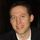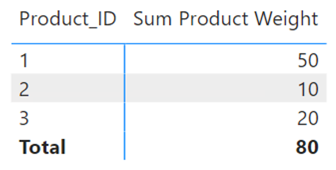cancel
Showing results for
Did you mean:Helper I

## SUM based on distinct values ​​from another column

Hello 🙂

I have a table in the following format:

 Product_ID Weight Classification 1 50 A 1 50 B 2 10 A 2 10 C 2 10 C 3 20 B

As you can see, the same product can be repeated several times in the table, but that product will always have the same weight.
I want to create a measure that makes a sum of the unique weights of each product based on the "Product_ID" column.

Based on the table above, this measurement would bring me the result: 80

because:

Product_ID 1 = 50
Product_ID 2 = 10
Product_ID 3 = 20

Can someone help me?

1 ACCEPTED SOLUTIONSuper User

Try this measure:

``````Sum Product Weight =
SUMX (
SUMMARIZE ( Table1, Table1[Product_ID], Table1[Weight] ),
Table1[Weight]
)``````Proud to be a Super User!Super User

Try this measure:

``````Sum Product Weight =
SUMX (
SUMMARIZE ( Table1, Table1[Product_ID], Table1[Weight] ),
Table1[Weight]
)``````Proud to be a Super User!